# How was the linear transformation of b1 and b2 were applied (L(b1) , L(b2))? NOTE: b1=(1,1)^T , b2=(-1,1)^T Linear Transformations EXAMPLE 4 Let L be a linear transformation mapping R? into itself an...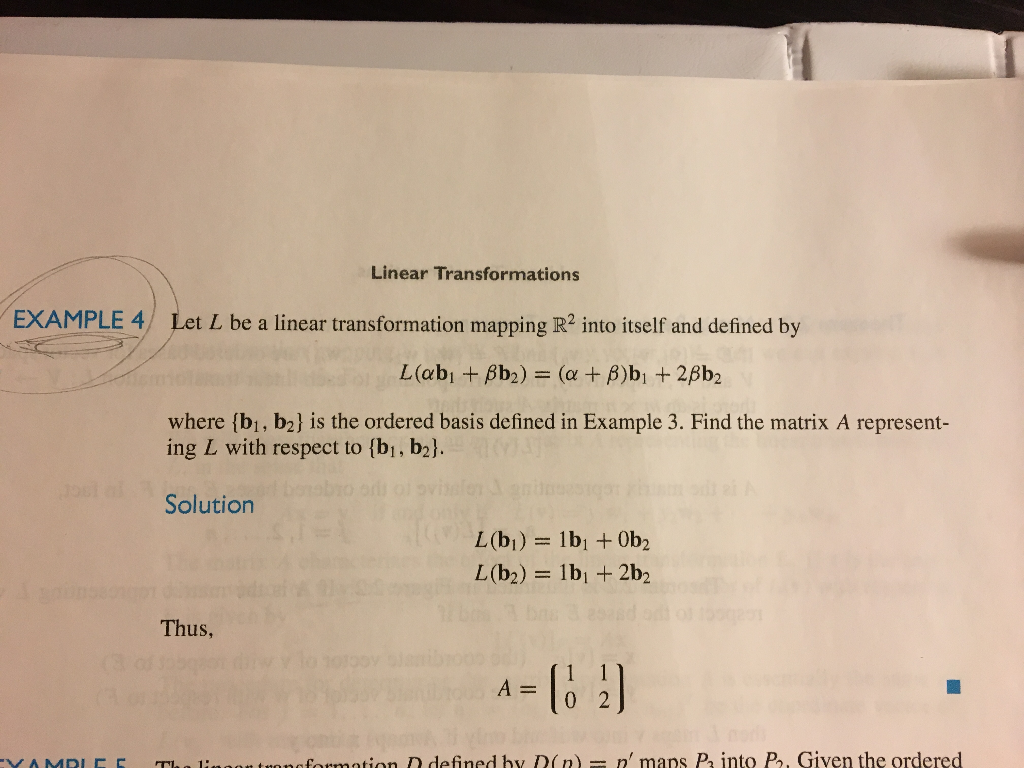How was the linear transformation of b1 and b2 were applied (L(b1) , L(b2))?

NOTE: b1=(1,1)^T , b2=(-1,1)^T

Linear Transformations EXAMPLE 4 Let L be a linear transformation mapping R? into itself and defined by where (bi, b2] is the ordered basis defined in Example 3. Find the matrix A represent- ing L with respect to [bi, b2l Solution Thus, A0 2 onofosmation D defined by D(n n' maps P into P, Given the ordered

I hope it was clear. Please comment for any further clarification.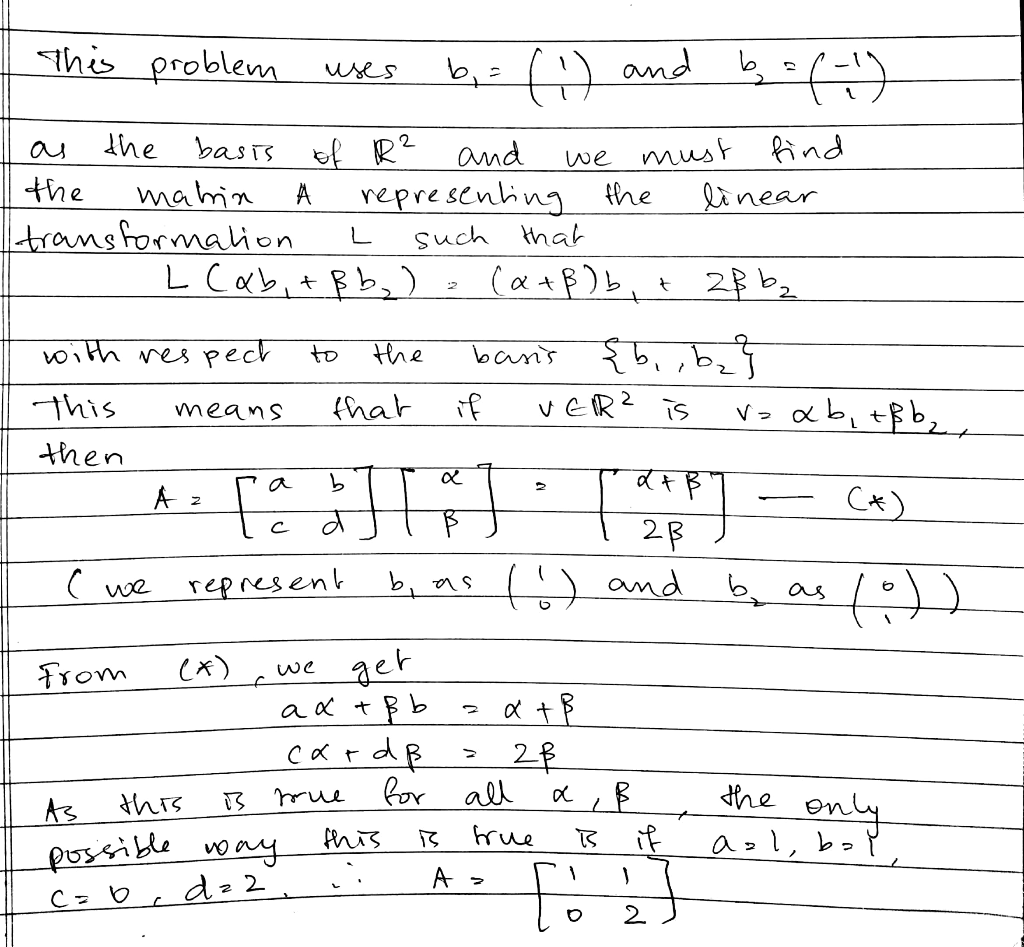#### Earn Coin

Coins can be redeemed for fabulous gifts.

Similar Homework Help Questions
• ### 3. This example hopes to illustrate why the vector spaces the linear transformation are defined o...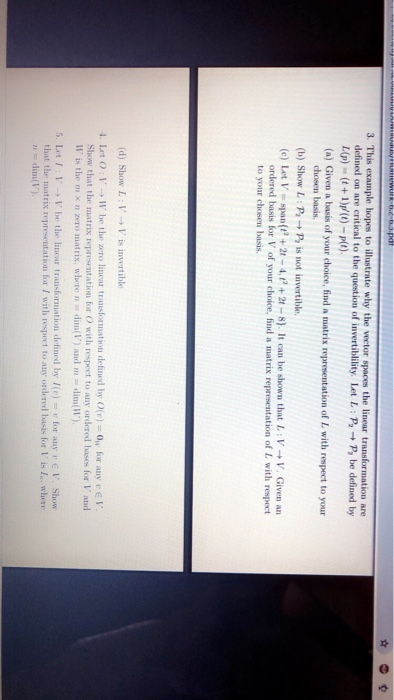3. This example hopes to illustrate why the vector spaces the linear transformation are defined on are critical to the question of invertibility. Let L : → p, be defined by L(p)(t+1)p(t)-plt). (a) Given a basis of your choice, find a matrix representation of I with respect to your chosen basis (b) Show L: P+P is not invertible (e) Let V-span+21-4,+2t-8). It can be shown that L VV. Given an ordered basis for V of your choice, find a matrix...

• ### 4) The linear transformation L defined by L(p(x)) = p'(x)+ p(0) maps P, into P. a)...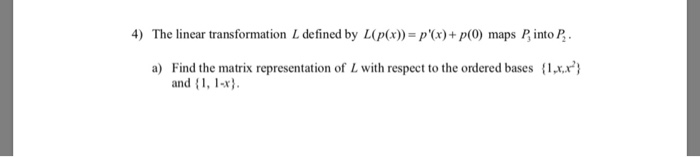4) The linear transformation L defined by L(p(x)) = p'(x)+ p(0) maps P, into P. a) Find the matrix representation of L with respect to the ordered bases {1xx.x"} and {1, 1-x} b) For the vector, p(x) = 2x2 + x-2 () find the coordinates of L(p(x)) with respect to the ordered basis {1, 1-x}., using the matrix you found in a). Remember to use the coordinate vector of p(x) with respect to the basis {1xx"}. (ii) Show that they...

• ### 4) The linear transformation L defined by L(p(x)) = p(x)+p(0) maps Pinto P. a) Find the...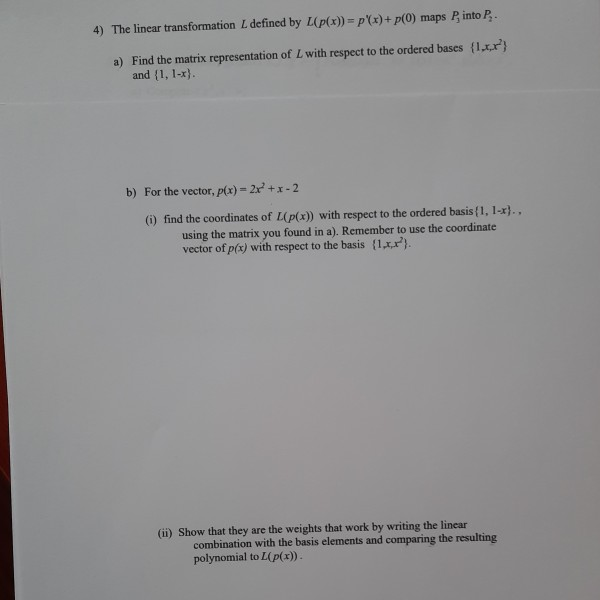4) The linear transformation L defined by L(p(x)) = p(x)+p(0) maps Pinto P. a) Find the matrix representation of L with respect to the ordered bases l_r"} and {1, 1-x). b) For the vector, p(x) = 2x' +1-2 () find the coordinates of L(p(x)) with respect to the ordered basis{1, 1-x), using the matrix you found in a). Remember to use the coordinate vector of p(x) with respect to the basis {1x2). (ii) Show that they are the weights that...

• ### Font Styles Paragraph Definition 1: Given La linear transformation from a vector space V into itself,...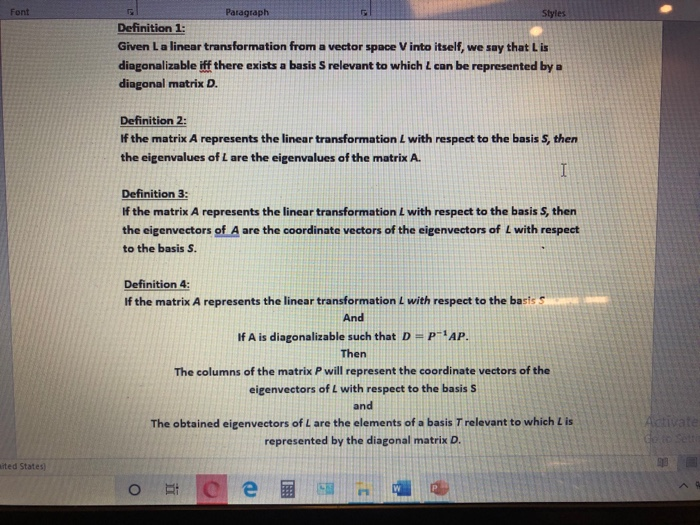Font Styles Paragraph Definition 1: Given La linear transformation from a vector space V into itself, we say that is diagonalizable iff there exists a basis S relevant to which can be represented by a diagonal matrix D. Definition 2: If the matrix A represents the linear transformation L with respect to the basis S, then the eigenvalues of L are the eigenvalues of the matrix A. I Definition 3: If the matrix A represents the linear transformation L with...

• ### 4) The linear transformation L defined by L(p(x)) = p'(x)+p(0) maps Pinto P. a) Find the...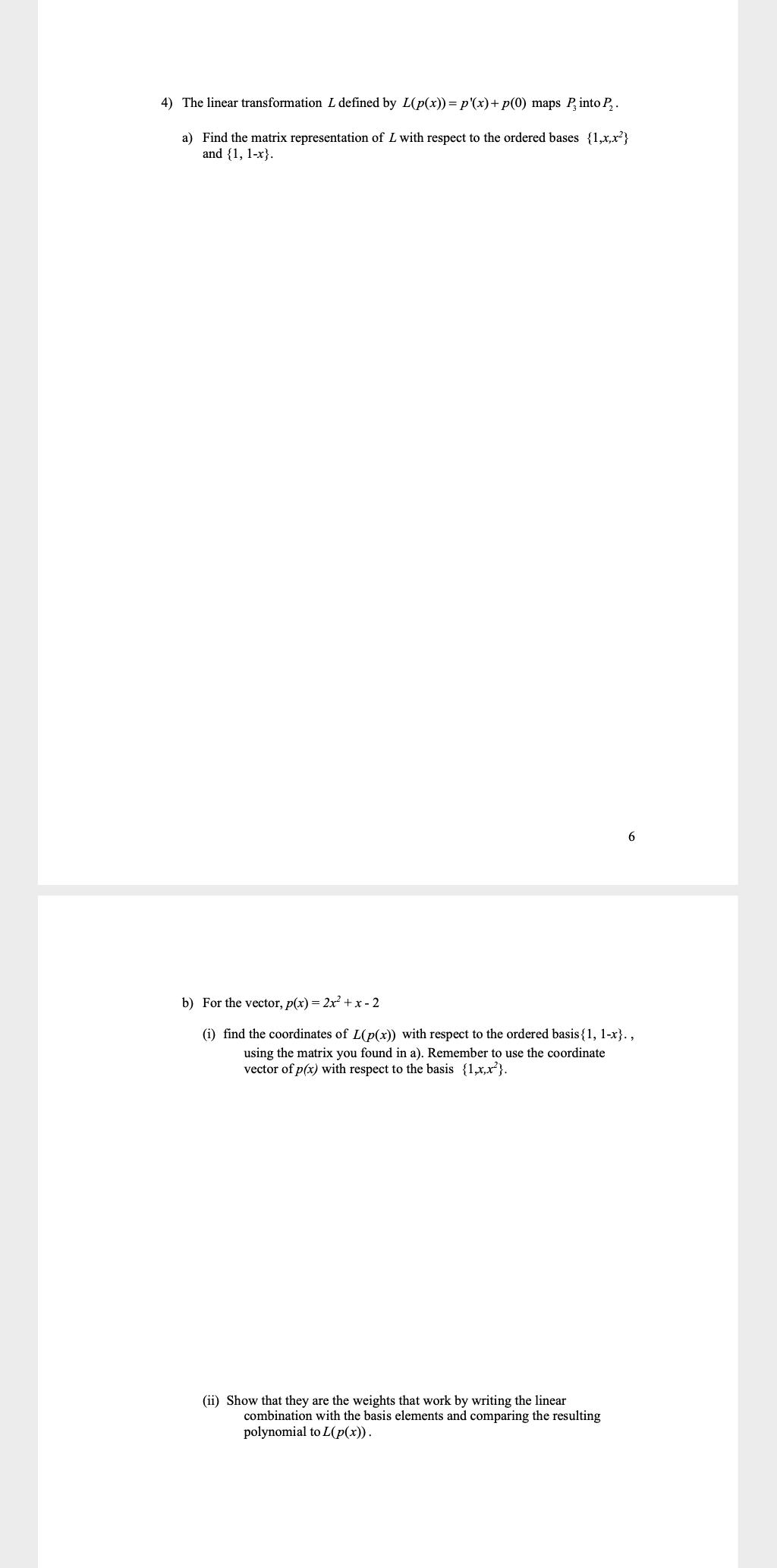4) The linear transformation L defined by L(p(x)) = p'(x)+p(0) maps Pinto P. a) Find the matrix representation of L with respect to the ordered bases {1,x,x} and {1, 1-x}. 6 b) For the vector, p(x) = 2x + x - 2 (i) find the coordinates of L(p(x)) with respect to the ordered basis{1, 1-x}. , using the matrix you found in a). Remember to use the coordinate vector of p(x) with respect to the basis {1,x,x"}. (ii) Show that...

• ### 2. Let b(1,-1,1). Define T: R3R3 by the mapping: T(x) (x b)b (a) Show that T is a linear transformation by verifying the two linear transformation axioms (b) Determine the standard matrix representat...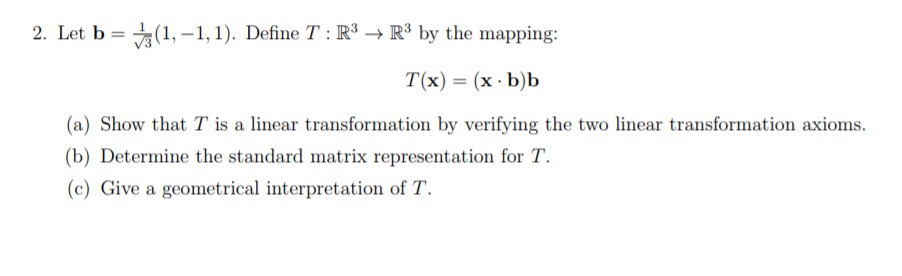2. Let b(1,-1,1). Define T: R3R3 by the mapping: T(x) (x b)b (a) Show that T is a linear transformation by verifying the two linear transformation axioms (b) Determine the standard matrix representation for T. (c) Give a geometrical interpretation of T. 2. Let b(1,-1,1). Define T: R3R3 by the mapping: T(x) (x b)b (a) Show that T is a linear transformation by verifying the two linear transformation axioms (b) Determine the standard matrix representation for T. (c) Give a...

• ### 1. Let L: P1(R) + P1(R) be a linear transformation given by L(a + bx) =...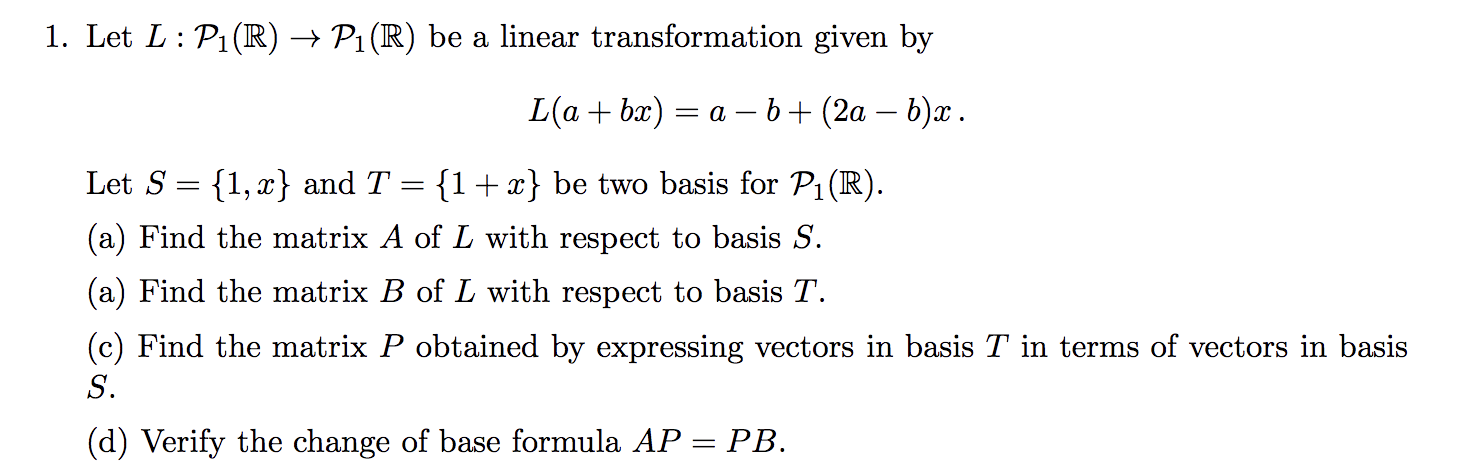1. Let L: P1(R) + P1(R) be a linear transformation given by L(a + bx) = a - b + (2a – b)x. Let S = {1, 2} and T = {1+x} be two basis for P1(R). (a) Find the matrix A of L with respect to basis S. (a) Find the matrix B of L with respect to basis T. (c) Find the matrix P obtained by expressing vectors in basis T in terms of vectors in basis (d)...

• ### 0 1 Let S span 1 1 1 0 }, a basis for S. Show that| (a) Let B1 { 1 0 1 1 0 is also a basis for S 0 B2 { 1 (b) Write eac...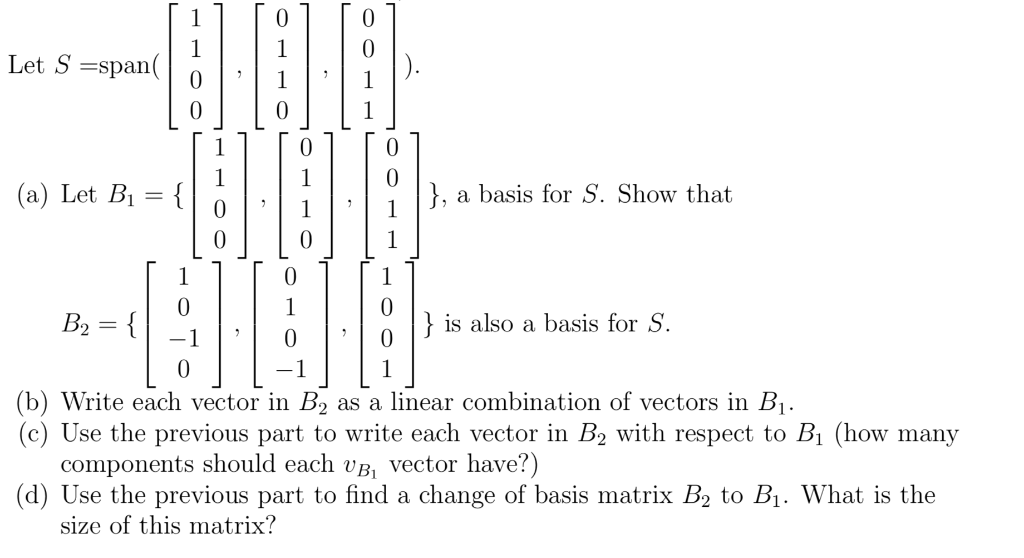0 1 Let S span 1 1 1 0 }, a basis for S. Show that| (a) Let B1 { 1 0 1 1 0 is also a basis for S 0 B2 { 1 (b) Write each vector in B2 (c) Use the previous part to write each vector in B2 with respect to Bi (how many components should each vB, vector have?) (d) Use the previous part to find a change of basis matrix B2 to B1. What...

• ### 6. Let L be the linear operator mapping R3 into R3 defined by L(x) Ax, where...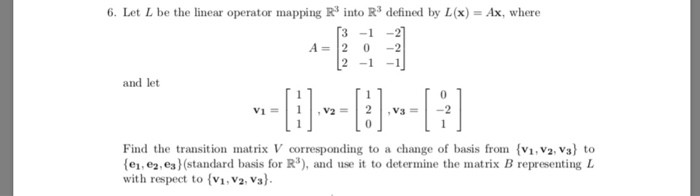6. Let L be the linear operator mapping R3 into R3 defined by L(x) Ax, where A=12 0-2 and let 0 0 Find the transition matrix V corresponding to a change of basis from i,V2. vs) to e,e,es(standard basis for R3), and use it to determine the matrix B representing L with respect to (vi, V2. V

• ### could u help me for this question?thanku!! 21. Let T be a linear transformation from P2 into P3 over R defined by T(p(x)) xp(x). (a) Find [T]B.A the matrix of T relative to the bases A = {1-x, l-x...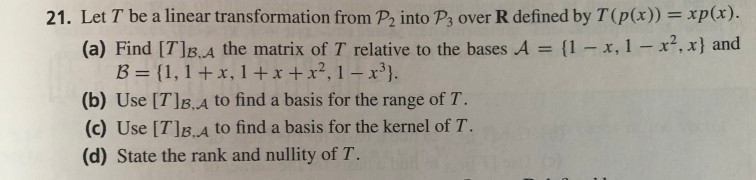could u help me for this question?thanku!! 21. Let T be a linear transformation from P2 into P3 over R defined by T(p(x)) xp(x). (a) Find [T]B.A the matrix of T relative to the bases A = {1-x, l-x2,x) and B={1,1+x, 1 +x+12, 1-x3}. (b) Use [TlB. A to find a basis for the range of T. (c) Use TB.A to find a basis for the kernel of T. (d) State the rank and nullity of T. 21. Let T...